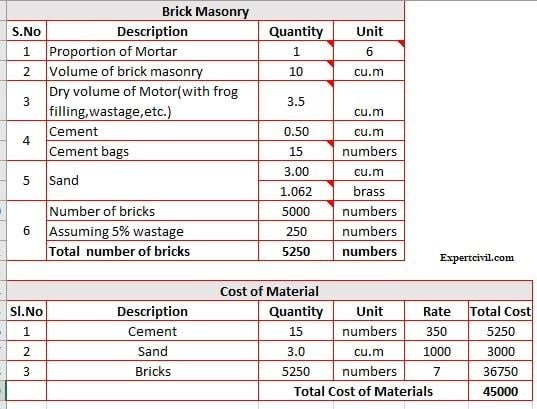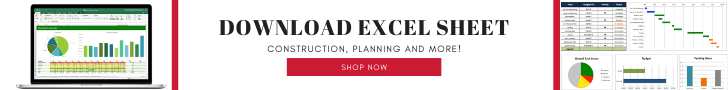Hello Dear friends welcome back to expertcivil.com’s blog so finally today I’m going to share “Calculation of Bricks Excel Sheet for Download”

Actually, I’m already shared about Different properties of bricks, Manufacturing of bricks, Classification of bricks and Test on bricks that be covered in the previous post.### Basics Concept of Brickwork

Here are given some basic points,

• Ensure to read the brickwork construction procedure provided by the client
• Cement mortar ratio should be 1:6 ratio for 9-inch Brickwork and 1:4 ratio for 4& 1/2” inch brickwork.
• The thickness of the mortar should not be more than 10 mm between the courses and sides of the bricks.
• Ensure you are using good quality of cement and sand.
• Ensure you have available all the Civil Engineering tools which are required for the construction.

### Volume of bricks with mortar

Volume of 1 brick with mortar = 200 X 100 X 100 ( 10 mm mortar thickness on all sides)

= 0.2  X 0.1 X 0.1

Volume of brick with mortar = 0.002 Cum (m3)

Therefore,

Number of bricks required for 1 cubic metre = 1/0.002

= 500 Numbers

### Volume of bricks without mortar

Volume of 1 brick without mortar = 190 X 90 X 90

= 0.19 X 0.09 X 0.09

Volume of 1 brick without mortar  =  0.001539 Cum (m3)

Volume of 500 bricks without mortar = 500 X 0.001539 Cum

Volume of bricks without mortar for 1 cum = 0.7695 Cum  (m3)

Therefore,

Required amount of cement mortar =

1 Cum – Volume of bricks without mortar = 1 – 0.7695

The required amount of cement mortar  = 0.2305 Cum  (m3) (Wet Condition)

Please Note – The above volume is in a wet condition that means we need 0.2305 cement mortar in mixed condition (after adding water). In order to find the dry volume, we need to multiply 33 % as bulkage of sand.

Dry volume of a mortar = 0.2305 cum X 1.33 = 0.306565 cum

As we know the mortar ratio is 1:6 (it means 1 part Cement & 6 Part Sand = 7 Part)

Required amount Cement quantity in brickwork = 0.306565 X 1/7 X 1440 kg

Density of cement = 1440 kg. The reason to multiply this density is, the above multiplication will give us only required amount of cement quantity in brickwork as a cubic metre. But we need cement in Kg. Therefore we are multiplying the 1440 kg density of cement to calculate the cement quantity.

Required amount Cement quantity = 63 Kg = 1.26 bags (50 Kg bag)

Required amount of Sand = 0.306565 X 6/7 = 0.26277 Cubic metre  (m3)

Therefore, For 1 cum of brickwork, we need

• 500 Numbers of bricks
• 63 kg of cement
• 0.263 mof sand.

You can also calculate Through given excel sheet (Calculation of Bricks Excel Sheet )

### Advantages of using Calculation of Brick’s Excel Sheet

1.  Easy to use & calculate numbers of bricks and cost of material.
2. The exact value of calculation.
3.  Easy to handle and rapid method for calculating the numbers of bricks.
4. You can also calculate the cost of materials
5. Time-saving.
6. No need technical skills and knowledge.1.